## Cubes and Dices

#### Cubes and Dices

1. From the following two different appearance of a dice, which number lies to the opposite of 2?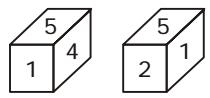1. According to the rule ( 1 ) of dice , we know that
Two opposite faces cannot be adjacent to one another.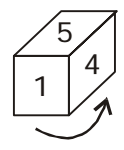##### Correct Option: B

According to the rule ( 1 ) of dice , we know that
Two opposite faces cannot be adjacent to one another.
Clearly, the number 4 lies to the opposite of 2.1. Two positions of a dice are given. Which number would be at the top when bottom is 2?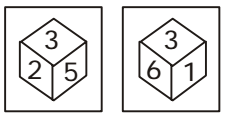1. On the basis of question figure pattern ,
The numbers 1, 2, 5 and 6 are on the adjacent faces of number 3.
Therefore, the number 4 lies opposite 3.
The numbers 3, 4 and 6 cannot be on the faces opposite to 1.

##### Correct Option: D

On the basis of question figure pattern ,
The numbers 1, 2, 5 and 6 are on the adjacent faces of number 3.
Therefore, the number 4 lies opposite 3.
The numbers 3, 4 and 6 cannot be on the faces opposite to 1.
Therefore, 5 lies opposite 1.
Now, 2 lies opposite 6.

1. Four positions of a dice are given below. Identify the number at the bottom when top is 6.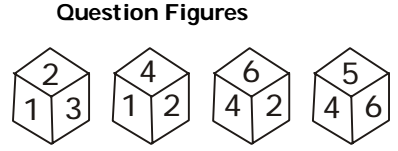1. On the basis of question figure pattern , we can see that
The numbers 1, 2, 5 and 6 are on the faces adjacent to the number 4.
So, the number 3 lies opposite 4.
The numbers 1, 3, 4 and 6 lies on the faces adjacent to the number 2.

##### Correct Option: A

On the basis of question figure pattern , we can see that
The numbers 1, 2, 5 and 6 are on the faces adjacent to the number 4.
So, the number 3 lies opposite 4.
The numbers 1, 3, 4 and 6 lies on the faces adjacent to the number 2.
So, the number 5 lies opposite 2.
Now, the number 1 lies opposite 6.

1. Four positions of a dice are given below. Identify the number at the bottom when the number on the top is 2.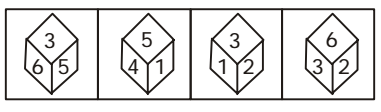1. On the basis of question figure pattern ,
The numbers 1, 2, 5 and 6 are on the faces adjacent to the number 3.
Therefore, 4 lies opposite 3.
The numbers 2, 3, 4 and 5 are on the faces adjacent to the number 1.

##### Correct Option: D

On the basis of question figure pattern ,
The numbers 1, 2, 5 and 6 are on the faces adjacent to the number 3.
Therefore, 4 lies opposite 3.
The numbers 2, 3, 4 and 5 are on the faces adjacent to the number 1.
Therefore, 6 lies opposite 1.
Now, the number 5 lies opposite 2.

1. Four positions of a dice are given below:
Find the number on the face opposite to the face showing 4.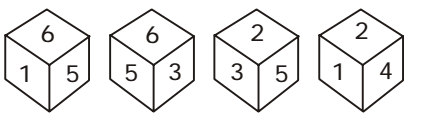1. As we can see that ,
The numbers 1, 2, 3 and 6 lie on the faces adjacent to the number 5.

##### Correct Option: A

As we can see that ,
The numbers 1, 2, 3 and 6 lie on the faces adjacent to the number 5.
Therefore, the number 5 lies opposite 4.